### Home > CALC > Chapter 9 > Lesson 9.4.3 > Problem9-135

9-135.
1. Examine the integrals below. Consider the multiple tools available for integrating and use the best strategy. After evaluating each integral, write a short description of your method. Homework Help ✎

1.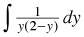2.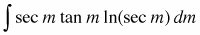3.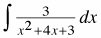4.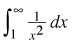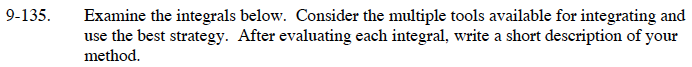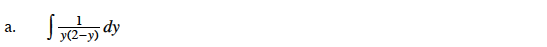Use partial fraction decomposition to rewrite the integrand.

$\frac{1}{y(2-y)}=\frac{a}{y}+\frac{b}{2-y}$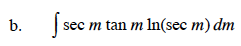Use substitution. Let u = sec(m).

du = sec(m)tan(m)dm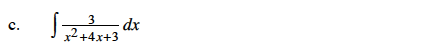Use partial fraction decomposition to rewrite the integrand.

$\frac{3}{x^2+4x+3}=\frac{a}{x+3}+\frac{b}{x+1}$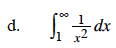This is an improper integral. Use a limit to properly evaluate this integral.